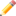This article is a stub. You can help Programmer's Wiki by expanding it.Remember to remove this when the stub is fixed.

In computer science, an array is a basic list of objects that occupies a contiguous area of memory in a computer (thus making it the most basic randomly accessible list).

Arrays are a built-in data type in almost all programming languages. The notation most often used is A[i], where A is the name of the array, and i is an index, usually ranging from 0 to n-1 (n being the number of elements in A).

Arrays are almost always implemented as a series of equally-sized memory locations. Because of this, the address of A[i] can be calculated in constant time. Another consequence of this implementation is that expanding an array can result in the entire array needing to be reallocated and copied, as it cannot be guaranteed that the address of A[n] is not allocated to another object. Some programming languages have built-in support for arrays that can be allocated and resized on the fly, while other programming languages (notably, C and C++) only support fixed-length arrays natively. An array is fixed-length if its length must be calculated at compile time. In C and C++, dynamic arrays can be implemented in library or application code (for example, with C++'s vector class).

The downsides of arrays are that the insertion and the remove operation are very slow (need linear time). A list is a alternative data structure which doesn't have such an disadvantage. A disadvantage of lists is that the indexing operation is slow. C++ Arrays

Many programming languages implement strings as arrays of characters. An array can also contain other arrays. This is known as a multi-dimensional array and provides a basic way to store data in a grid.

## PHP

In some programming languages, such as PHP, arrays are implemented as hash tables. As a result, access to the elements of these arrays takes longer than constant time:

```/* define array using auto-generated keys */
\$data = array('apple', 'orange');

/* show values */
print_r(\$data);
#Array
#(
#     => apple
#     => orange
#)

/* define array with user-defined keys */
\$data = array('a'=>'apple', 'o'=>'orange');

/* show values */
print_r(\$data);
#Array
#(
#    [a] => apple
#    [o] => orange
#)
```

## D

Some programming languages support two different kinds of array: Associative arrays (hash tables) and indexed arrays (the type of array supported by most other programming languages):

```int main()
{
int[char[]] assoc; // A hash table with strings for keys.
int indexed;    // A fixed-length array.

assoc["foobar"] = 3.14;
indexed = assoc["foobar"] + 0.0059;
writefln("Less precise: %f More precise: %f", assoc["foobar"], indexed);
return 0;
}
```

## Java

```// define a class named array
class array {
// define main method of this java code
public static void main(String[] args){

// declare an array named intArray for integer type values
int[] intArray = {14,15,16,17,18};
for (int i = 0; i < intArray.length; i++) {
System.out.println((i+1) + " Element of array : " + intArray[i]);
}
}
}
```

## C++

```#include <cstdlib>
#include <iostream>
#include <vector>
#include <string>
int main(int argc, char* argv[]) {
// declare an array named intArray for integer type values on the stack
int iStackArray[] = {14,15,16,17,18};
for(int i=0; i<5; i++) {
std::cout << (i+1) << " Element of array : " << iStackArray[i] << std::endl;
}
// declare an empty array of char with a size of 15 on the heap
// In c and c++ arrays are pointers to contiguous memory locations
// and is not abstracted away from the programmer.
char* cHeapArray = new char;

// declate an empty array of string with a size variable size using STL.
std::vector<std::string> sVectorArray;

// if the cHeapArray is not needed anymore, free it by calling delete[] (not delete)!!!
delete[] cHeapArray;

return(EXIT_SUCCESS);
}
```